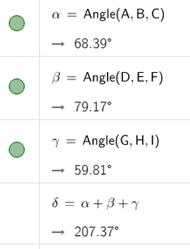# Doing calculations

msaul shared this question 2 years ago

I have a diagram with angles alpha, beta, gamma. I want to show the sum of the three measures of the three angles. I want the sum to change dynamically as the sketch changes. How do I do this?

I can't seem to enter a measurement from the sketch into the spreadsheet.

I can't find this simple thing for the life of me in documentation!1

To write to Cell A1

A1=α+β+γ

really necessary to use the spreadsheet?1

Something is missing from this answer. I don't want the *symbols \alpha + \beta + \gamma in the spreadsheet--which is what I get when I follow these instructions. I want the sum of the measures of three angles.

But I don't care if it's not in the spreadsheet. Geogebra *must* have a tool to compute with measurements. How do I do it? The tutorials don't answer that. The examples given are not what I want. The 'help' files--this is typical of most software--don't really help.1

an answer can only use the information given, quite mysterious where the problem is to add 3 angles

i intend the symbols \alpha + \beta + \gamma are variables of the angles

it is a common use to upload an appropriate file, this is what i am missing1

Can you just type this?

α+β+γ1